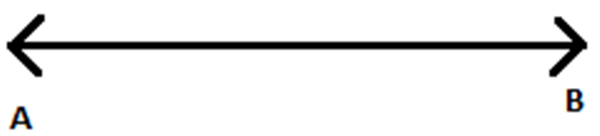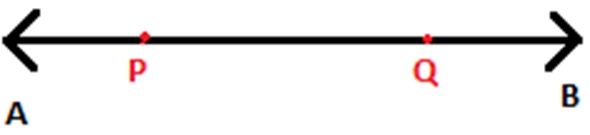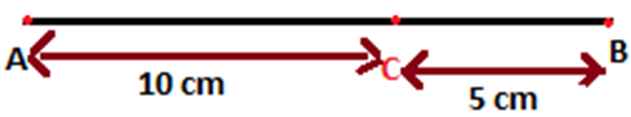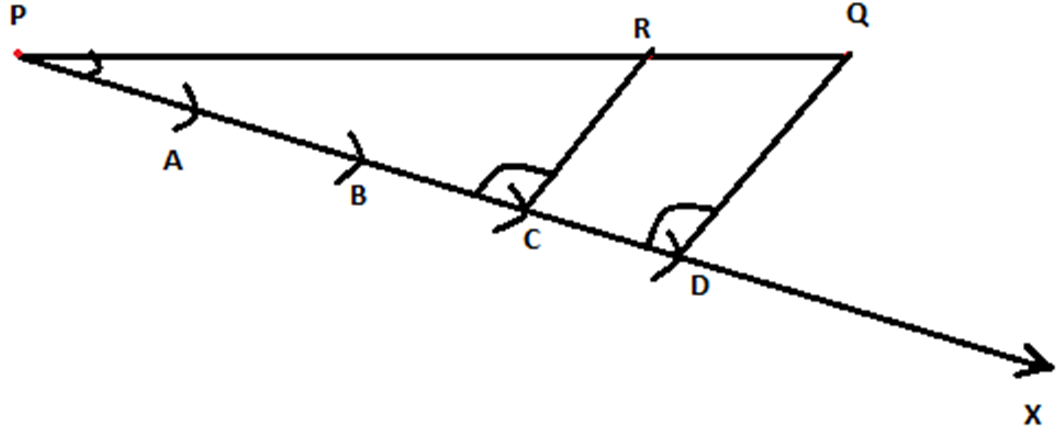# Division Of A Line Segment

Before knowing about the division of a Line segment, let us know what a line and line segment means,

Line and line segment

A line is a collection of points along a straight path which extends to both the directions without endpoints.AB is a line which doesn’t have an ending.A line segment is a part of a line between two endpoints.

PQ is a line segment having P and Q as endpoints on the line AB.

## Division of a line segment Definition

A line segment can be divided into ‘n’ equal parts, where ‘n’ is any natural number.

For example; a line segment of length 10 cm is divided into two equal parts by using a ruler as,

• Mark a point 5 cm away from one end.
• 10 cm is divided into two 5 cm line segments.

Similarly, a line segment of length 15 cm can be divided in the ratio 2:1 as,

• AB is the line segment of length 15 cm and C divides the line in the ratio 2:1.
• Let CB = x, then AC = 2x
• AC + CB = 2x + x = 15, x = 5
• AC = 10 cm and CB = 5 cm.
• Mark point C, 10 cm away from ANow, what if we cannot measure the lengths precisely? We will not be able to mark the point correctly.

There is a better way to mark point while dividing a line in a given ratio, which explained as follows.

Consider a line

$$\begin{array}{l} \overleftrightarrow{PQ}\end{array}$$
. We have to divide
$$\begin{array}{l} \overleftrightarrow{PQ}\end{array}$$
in a ratio m:n, where m and n are positive integers.

Let m = 3 and n = 1. So, we are dividing the line

$$\begin{array}{l} \overleftrightarrow{PQ}\end{array}$$
in the ratio 3 : 1.

### Steps of construction

• Draw
$$\begin{array}{l}\overleftrightarrow{PQ}\end{array}$$
a ray PX which makes acute angle with
$$\begin{array}{l} \overleftrightarrow{PQ}\end{array}$$
• Since the given ratio is 3:1, the number of points to be located on PX should be 4. Because(m + n = 3 + 1) . Now mark the points A, B, C and D such that PA = AB = BC = CD using compass. Draw an arc with a measure of n/4, from the centre P and name it as A. Drawing another arc by taking the centre as the first marked arc (A), draw an arc with the same measure and name it as B. Similarly, do it for other two arcs, also. (For example, if the measure of the line segment pQ = 20 cm, divide 20 by 4, we will get 5. Now, take the compass and measure 5 cm. Take P as a centre and draw an arc on PX and mark that point as A. Now take A as a centre and draw another arc on the same ray PX and mark it as B. Similarly, repeat this process for 2 more times and mark it as C and D).
• Join Q and D using a ruler.
• Draw a line through the point C (m = 3, C is the third point from P) which should be parallel to
$$\begin{array}{l} \overleftrightarrow{QD}\end{array}$$
by making an equal angle to ∠PDQ intersect the line PQ at R. (Refer figure.)
• Now, R is the point on
$$\begin{array}{l} \overleftrightarrow{PQ}\end{array}$$
⃡which divides
$$\begin{array}{l} \overleftrightarrow{PQ}\end{array}$$
in the ratio 3:1.Figure 1-Division of a line segment

Since CR is parallel to DQ;

By basic proportionality theorem,

$$\begin{array}{l} \frac {PR}{RQ}\end{array}$$
=
$$\begin{array}{l} \frac {PC}{CD}\end{array}$$

By construction,

$$\begin{array}{l} \frac {PC}{CD}\end{array}$$
=
$$\begin{array}{l} \frac {3}{1}\end{array}$$

Therefore,

$$\begin{array}{l} \frac {PR}{RQ}\end{array}$$
=
$$\begin{array}{l} \frac {3}{1}\end{array}$$

To watch interesting videos on the topic, download BYJU’S – The Learning App from Google Play Store.